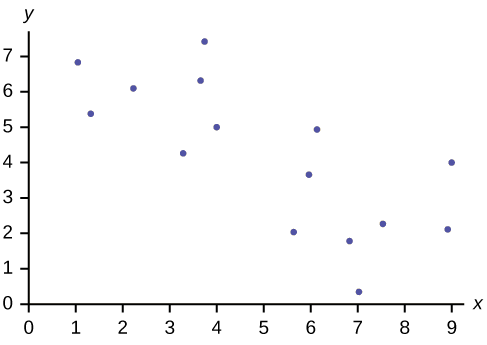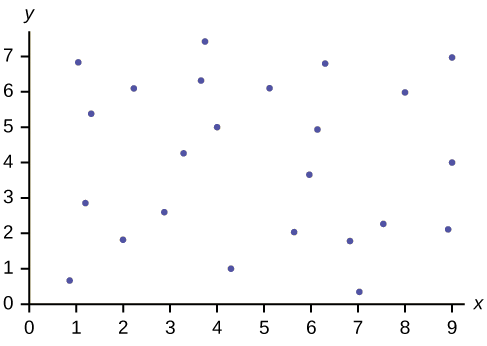# 12.3E: Scatter Plots (Exercises)

Exercise $$\PageIndex{1}$$

Does the scatter plot appear linear? Strong or weak? Positive or negative?Figure $$\PageIndex{4}$$

The data appear to be linear with a strong, positive correlation.

Exercise $$\PageIndex{3}$$

Does the scatter plot appear linear? Strong or weak? Positive or negative?Figure $$\PageIndex{5}$$

Exercise $$\PageIndex{4}$$

Does the scatter plot appear linear? Strong or weak? Positive or negative?Figure $$\PageIndex{6}$$